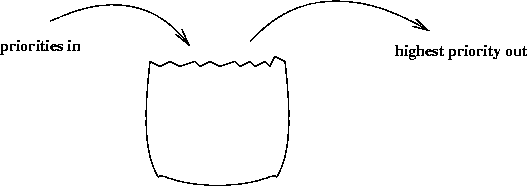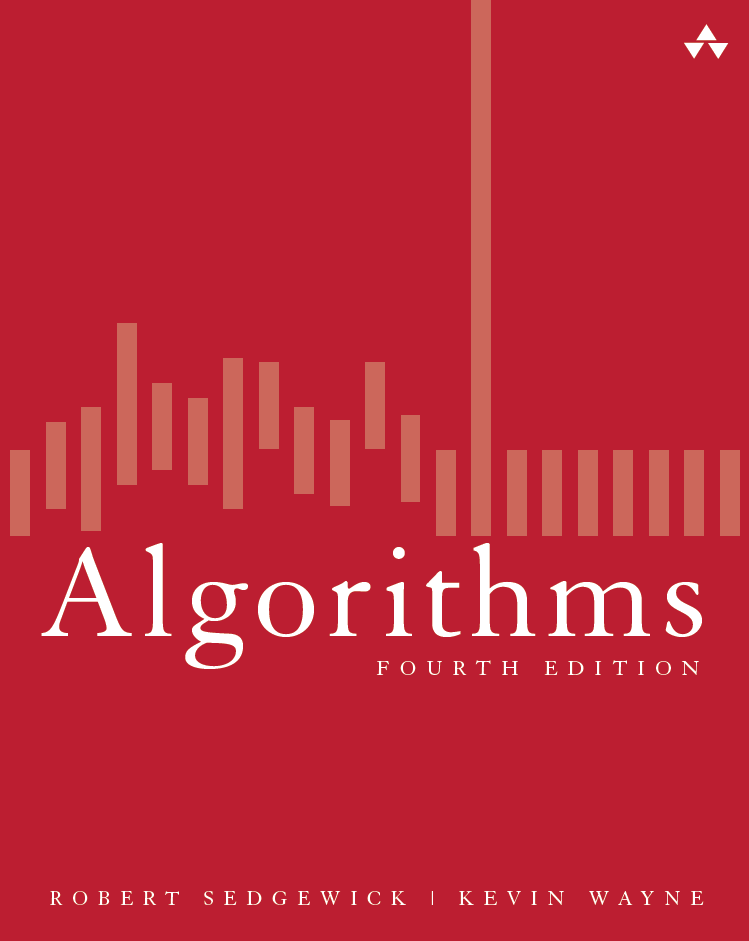Algorithms and Time Complexity This group is for discussions related to Algorithms and their Time Complexity
 Priority QueuesPriority QueuesIntroductionHeaps 06-Jan-2014
 Priority QueuesThe textbook Algorithms, 4th Edition by Robert Sedgewick and Kevin Wayne surveys the most important algorithms and data structures in use today. The broad perspective taken makes it an appropriate introduction to the field. 06-Jan-2014
 Big O notation - Wikipedia, the free encyclopediaBig O notation : In mathematics, big O notation describes the limiting behavior of a function when the argument tends towards a particular value or infinity, usually in terms of simpler functions. It is a member of a larger family of notations that is called Landau notation, Bachmann?Landau notation (after Edmund Landau and Paul Bachmann), or asymptotic notation. In computer science, big O notation is used to classify algorithms by how they respond (e.g., in their processing time or working space requirements) to changes in input size. In analytic number theory, it is used to estimate the "error committed" while replacing the asymptotic size, or asymptotic mean size, of an arithmetical function, by the value, or mean value, it takes at a large finite argument. A famous example is the problem of estimating the remainder term in the prime number theorem. 29-Sep-2013
 Binary Search TreesThe textbook Algorithms, 4th Edition by Robert Sedgewick and Kevin Wayne surveys the most important algorithms and data structures in use today. The broad perspective taken makes it an appropriate introduction to the field. 25-Sep-2013
Search Results fromNext>>